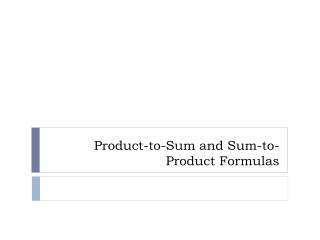DownloadDownload PresentationProduct-to-Sum and Sum-to-Product Formulas

# Product-to-Sum and Sum-to-Product Formulas

Télécharger la présentation## Product-to-Sum and Sum-to-Product Formulas

- - - - - - - - - - - - - - - - - - - - - - - - - - - E N D - - - - - - - - - - - - - - - - - - - - - - - - - - -
##### Presentation Transcript

1. Product-to-Sum and Sum-to-Product Formulas

2. Product-to-Sum Formulas • Changing an equation from a multiplication problem to an addition problem

3. Expressing Products as Sums • Express each of the following products as a sum containing only sines or cosines. • (a) sin (6q) sin (4q) • (b) cos (3q) cos (q)

4. Expressing Products as Sums • (c) sin (3q) cos (5q)

5. Sum-to-Product Formulas • Changing equations from an addition problem to a multiplication problem

6. Expressing Sums as a Product • Express each sum or difference as a product of sines and/or cosines. • (a) sin (5q) – sin (3q) • (b) sin (4q) + sin (2q)

7. Expressing Sums as Products • (c) cos q – cos (3q) • (d) cos (5q) + cos (3q)

8. Establishing Identities • Establish the identity

9. Establishing Identities • Establish the identity

10. Product-to-Sum and Sum-to-Product • On-line Tutorial • On-line Video